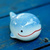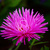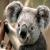# RSA算法，为什么我将加密后的 byte[] 数组 传给前端，前端回传给我后，解密失败呢

javacc 发布于 2018/08/22 18:57

0byte[]与String之间前后端是否用了相同的编码？一般最好统一使用UTF-8

000#### 引用来自“节节草”的评论

byte[]与String之间前后端是否用了相同的编码？一般最好统一使用UTF-8

0``````package com.yueque.util;

import org.slf4j.Logger;
import org.slf4j.LoggerFactory;

/**
* @author
*/
public class Base64 {
private static Logger logger = LoggerFactory.getLogger(Base64.class);

static private final int     BASELENGTH           = 128;
static private final int     LOOKUPLENGTH         = 64;
static private final int     TWENTYFOURBITGROUP   = 24;
static private final int     EIGHTBIT             = 8;
static private final int     SIXTEENBIT           = 16;
static private final int     FOURBYTE             = 4;
static private final int     SIGN                 = -128;
static private final char    PAD                  = '=';
static private final boolean fDebug               = false;
static final private byte[]  base64Alphabet       = new byte[BASELENGTH];
static final private char[]  lookUpBase64Alphabet = new char[LOOKUPLENGTH];

static {
for (int i = 0; i < BASELENGTH; ++i) {
base64Alphabet[i] = -1;
}
for (int i = 'Z'; i >= 'A'; i--) {
base64Alphabet[i] = (byte) (i - 'A');
}
for (int i = 'z'; i >= 'a'; i--) {
base64Alphabet[i] = (byte) (i - 'a' + 26);
}

for (int i = '9'; i >= '0'; i--) {
base64Alphabet[i] = (byte) (i - '0' + 52);
}

base64Alphabet['+'] = 62;
base64Alphabet['/'] = 63;

for (int i = 0; i <= 25; i++) {
lookUpBase64Alphabet[i] = (char) ('A' + i);
}

for (int i = 26, j = 0; i <= 51; i++, j++) {
lookUpBase64Alphabet[i] = (char) ('a' + j);
}

for (int i = 52, j = 0; i <= 61; i++, j++) {
lookUpBase64Alphabet[i] = (char) ('0' + j);
}
lookUpBase64Alphabet = (char) '+';
lookUpBase64Alphabet = (char) '/';

}

private static boolean isWhiteSpace(char octect) {
return (octect == 0x20 || octect == 0xd || octect == 0xa || octect == 0x9);
}

private static boolean isPad(char octect) {
}

private static boolean isData(char octect) {
return (octect < BASELENGTH && base64Alphabet[octect] != -1);
}

/**
* Encodes hex octects into Base64
*
* @param binaryData Array containing binaryData
* @return Encoded Base64 array
*/
public static String encode(byte[] binaryData) {

if (binaryData == null) {
return null;
}

int lengthDataBits = binaryData.length * EIGHTBIT;
if (lengthDataBits == 0) {
return "";
}

int fewerThan24bits = lengthDataBits % TWENTYFOURBITGROUP;
int numberTriplets = lengthDataBits / TWENTYFOURBITGROUP;
int numberQuartet = fewerThan24bits != 0 ? numberTriplets + 1 : numberTriplets;
char encodedData[] = null;

encodedData = new char[numberQuartet * 4];

byte k = 0, l = 0, b1 = 0, b2 = 0, b3 = 0;

int encodedIndex = 0;
int dataIndex = 0;
if (fDebug) {
logger.info("number of triplets = " + numberTriplets);
}

for (int i = 0; i < numberTriplets; i++) {
b1 = binaryData[dataIndex++];
b2 = binaryData[dataIndex++];
b3 = binaryData[dataIndex++];

if (fDebug) {
logger.info("b1= " + b1 + ", b2= " + b2 + ", b3= " + b3);
}

l = (byte) (b2 & 0x0f);
k = (byte) (b1 & 0x03);

byte val1 = ((b1 & SIGN) == 0) ? (byte) (b1 >> 2) : (byte) ((b1) >> 2 ^ 0xc0);
byte val2 = ((b2 & SIGN) == 0) ? (byte) (b2 >> 4) : (byte) ((b2) >> 4 ^ 0xf0);
byte val3 = ((b3 & SIGN) == 0) ? (byte) (b3 >> 6) : (byte) ((b3) >> 6 ^ 0xfc);

if (fDebug) {
logger.info("val2 = " + val2);
logger.info("k4   = " + (k << 4));
logger.info("vak  = " + (val2 | (k << 4)));
}

encodedData[encodedIndex++] = lookUpBase64Alphabet[val1];
encodedData[encodedIndex++] = lookUpBase64Alphabet[val2 | (k << 4)];
encodedData[encodedIndex++] = lookUpBase64Alphabet[(l << 2) | val3];
encodedData[encodedIndex++] = lookUpBase64Alphabet[b3 & 0x3f];
}

// form integral number of 6-bit groups
if (fewerThan24bits == EIGHTBIT) {
b1 = binaryData[dataIndex];
k = (byte) (b1 & 0x03);
if (fDebug) {
logger.info("b1=" + b1);
logger.info("b1<<2 = " + (b1 >> 2));
}
byte val1 = ((b1 & SIGN) == 0) ? (byte) (b1 >> 2) : (byte) ((b1) >> 2 ^ 0xc0);
encodedData[encodedIndex++] = lookUpBase64Alphabet[val1];
encodedData[encodedIndex++] = lookUpBase64Alphabet[k << 4];
} else if (fewerThan24bits == SIXTEENBIT) {
b1 = binaryData[dataIndex];
b2 = binaryData[dataIndex + 1];
l = (byte) (b2 & 0x0f);
k = (byte) (b1 & 0x03);

byte val1 = ((b1 & SIGN) == 0) ? (byte) (b1 >> 2) : (byte) ((b1) >> 2 ^ 0xc0);
byte val2 = ((b2 & SIGN) == 0) ? (byte) (b2 >> 4) : (byte) ((b2) >> 4 ^ 0xf0);

encodedData[encodedIndex++] = lookUpBase64Alphabet[val1];
encodedData[encodedIndex++] = lookUpBase64Alphabet[val2 | (k << 4)];
encodedData[encodedIndex++] = lookUpBase64Alphabet[l << 2];
}

return new String(encodedData);
}

/**
* Decodes Base64 data into octects
*
* @param encoded string containing Base64 data
* @return Array containind decoded data.
*/
public static byte[] decode(String encoded) {

if (encoded == null) {
return null;
}

char[] base64Data = encoded.toCharArray();
// remove white spaces
int len = removeWhiteSpace(base64Data);

if (len % FOURBYTE != 0) {
return null;//should be divisible by four
}

int numberQuadruple = (len / FOURBYTE);

return new byte;
}

byte decodedData[] = null;
byte b1 = 0, b2 = 0, b3 = 0, b4 = 0;
char d1 = 0, d2 = 0, d3 = 0, d4 = 0;

int i = 0;
int encodedIndex = 0;
int dataIndex = 0;
decodedData = new byte[(numberQuadruple) * 3];

for (; i < numberQuadruple - 1; i++) {

if (!isData((d1 = base64Data[dataIndex++])) || !isData((d2 = base64Data[dataIndex++]))
|| !isData((d3 = base64Data[dataIndex++]))
|| !isData((d4 = base64Data[dataIndex++]))) {
return null;
}//if found "no data" just return null

b1 = base64Alphabet[d1];
b2 = base64Alphabet[d2];
b3 = base64Alphabet[d3];
b4 = base64Alphabet[d4];

decodedData[encodedIndex++] = (byte) (b1 << 2 | b2 >> 4);
decodedData[encodedIndex++] = (byte) (((b2 & 0xf) << 4) | ((b3 >> 2) & 0xf));
decodedData[encodedIndex++] = (byte) (b3 << 6 | b4);
}

if (!isData((d1 = base64Data[dataIndex++])) || !isData((d2 = base64Data[dataIndex++]))) {
return null;//if found "no data" just return null
}

b1 = base64Alphabet[d1];
b2 = base64Alphabet[d2];

d3 = base64Data[dataIndex++];
d4 = base64Data[dataIndex++];
if (!isData((d3)) || !isData((d4))) {//Check if they are PAD characters
if ((b2 & 0xf) != 0)//last 4 bits should be zero
{
return null;
}
byte[] tmp = new byte[i * 3 + 1];
System.arraycopy(decodedData, 0, tmp, 0, i * 3);
tmp[encodedIndex] = (byte) (b1 << 2 | b2 >> 4);
return tmp;
b3 = base64Alphabet[d3];
if ((b3 & 0x3) != 0)//last 2 bits should be zero
{
return null;
}
byte[] tmp = new byte[i * 3 + 2];
System.arraycopy(decodedData, 0, tmp, 0, i * 3);
tmp[encodedIndex++] = (byte) (b1 << 2 | b2 >> 4);
tmp[encodedIndex] = (byte) (((b2 & 0xf) << 4) | ((b3 >> 2) & 0xf));
return tmp;
} else {
return null;
}
} else { //No PAD e.g 3cQl
b3 = base64Alphabet[d3];
b4 = base64Alphabet[d4];
decodedData[encodedIndex++] = (byte) (b1 << 2 | b2 >> 4);
decodedData[encodedIndex++] = (byte) (((b2 & 0xf) << 4) | ((b3 >> 2) & 0xf));
decodedData[encodedIndex++] = (byte) (b3 << 6 | b4);

}

return decodedData;
}

/**
* remove WhiteSpace from MIME containing encoded Base64 data.
*
* @param data  the byte array of base64 data (with WS)
* @return      the new length
*/
private static int removeWhiteSpace(char[] data) {
if (data == null) {
return 0;
}

// count characters that's not whitespace
int newSize = 0;
int len = data.length;
for (int i = 0; i < len; i++) {
if (!isWhiteSpace(data[i])) {
data[newSize++] = data[i];
}
}
return newSize;
}
}
``````

``````package com.yueque.util;

import org.apache.commons.codec.binary.Base64;

import java.security.InvalidKeyException;
import java.security.KeyFactory;
import java.security.KeyPair;
import java.security.KeyPairGenerator;
import java.security.NoSuchAlgorithmException;
import java.security.PrivateKey;
import java.security.PublicKey;
import java.security.interfaces.RSAPrivateKey;
import java.security.interfaces.RSAPublicKey;
import java.security.spec.InvalidKeySpecException;
import java.security.spec.PKCS8EncodedKeySpec;
import java.security.spec.X509EncodedKeySpec;
import java.util.HashMap;
import java.util.Map;

import javax.crypto.Cipher;
import javax.crypto.IllegalBlockSizeException;

public class RSAUtils {
public static final String KEY_ALGORITHM = "RSA";
public static final String CIPHER_ALGORITHM = "RSA/ECB/PKCS1Padding";
public static final String PUBLIC_KEY = "publicKey";
public static final String PRIVATE_KEY = "privateKey";

/** RSA密钥长度必须是64的倍数，在512~65536之间。默认是1024 */
public static final int KEY_SIZE = 512;

public static final String PLAIN_TEXT = "123322";

public static void main(String[] args) {
Map<String, byte[]> keyMap = generateKeyBytes();

// 加密
PublicKey publicKey = restorePublicKey(keyMap.get(PUBLIC_KEY));

byte[] encodedText = RSAEncode(publicKey, PLAIN_TEXT.getBytes());
System.out.println("RSA encoded: " + Base64.encodeBase64String(encodedText));

// 解密
PrivateKey privateKey = restorePrivateKey(keyMap.get(PRIVATE_KEY));
System.out.println("RSA decoded: " + RSADecode(privateKey, encodedText));
}

/**
* 生成密钥对。注意这里是生成密钥对KeyPair，再由密钥对获取公私钥
*
* @return
*/
public static Map<String, byte[]> generateKeyBytes() {
try {
KeyPairGenerator keyPairGenerator = KeyPairGenerator
.getInstance(KEY_ALGORITHM);
keyPairGenerator.initialize(KEY_SIZE);
KeyPair keyPair = keyPairGenerator.generateKeyPair();
RSAPublicKey publicKey = (RSAPublicKey) keyPair.getPublic();
RSAPrivateKey privateKey = (RSAPrivateKey) keyPair.getPrivate();

Map<String, byte[]> keyMap = new HashMap<String, byte[]>();
keyMap.put(PUBLIC_KEY, publicKey.getEncoded());
keyMap.put(PRIVATE_KEY, privateKey.getEncoded());
return keyMap;
} catch (NoSuchAlgorithmException e) {
// TODO Auto-generated catch block
e.printStackTrace();
}
return null;
}

/**
* 还原公钥，X509EncodedKeySpec 用于构建公钥的规范
*
* @param keyBytes
* @return
*/
public static PublicKey restorePublicKey(byte[] keyBytes) {
X509EncodedKeySpec x509EncodedKeySpec = new X509EncodedKeySpec(keyBytes);

try {
KeyFactory factory = KeyFactory.getInstance(KEY_ALGORITHM);
PublicKey publicKey = factory.generatePublic(x509EncodedKeySpec);
return publicKey;
} catch (NoSuchAlgorithmException | InvalidKeySpecException e) {
// TODO Auto-generated catch block
e.printStackTrace();
}
return null;
}

/**
* 还原私钥，PKCS8EncodedKeySpec 用于构建私钥的规范
*
* @param keyBytes
* @return
*/
public static PrivateKey restorePrivateKey(byte[] keyBytes) {
PKCS8EncodedKeySpec pkcs8EncodedKeySpec = new PKCS8EncodedKeySpec(
keyBytes);
try {
KeyFactory factory = KeyFactory.getInstance(KEY_ALGORITHM);
PrivateKey privateKey = factory
.generatePrivate(pkcs8EncodedKeySpec);
return privateKey;
} catch (NoSuchAlgorithmException | InvalidKeySpecException e) {
// TODO Auto-generated catch block
e.printStackTrace();
}
return null;
}

/**
* 加密，三步走。
*
* @param key
* @param plainText
* @return
*/
public static byte[] RSAEncode(PublicKey key, byte[] plainText) {
try {
Cipher cipher = Cipher.getInstance(CIPHER_ALGORITHM);
cipher.init(Cipher.ENCRYPT_MODE, key);
return cipher.doFinal(plainText);
| InvalidKeyException | IllegalBlockSizeException
// TODO Auto-generated catch block
e.printStackTrace();
}
return null;
}

/**
* 解密，三步走。
*
* @param key
* @param encodedText
* @return
*/
public static String RSADecode(PrivateKey key, byte[] encodedText) {
try {
Cipher cipher = Cipher.getInstance(CIPHER_ALGORITHM);
cipher.init(Cipher.DECRYPT_MODE, key);
return new String(cipher.doFinal(encodedText));
| InvalidKeyException | IllegalBlockSizeException
// TODO Auto-generated catch block
e.printStackTrace();
}
return null;
}
}``````

``````Map<String, byte[]> keyMap = RSAUtils.generateKeyBytes();
PublicKey publicKey = RSAUtils.restorePublicKey(keyMap.get(RSAUtils.PUBLIC_KEY));
byte[] test = RSAUtils.RSAEncode(publicKey, obj.getMessage().getBytes("UTF-8"));
resultMap.put("iMessage", Base64.encode(test));``````

``byte[] iMessage = Base64.decode(request.getParameter("iMessage"));``

``RSAUtils.RSADecode(privateKey, iMessage)``